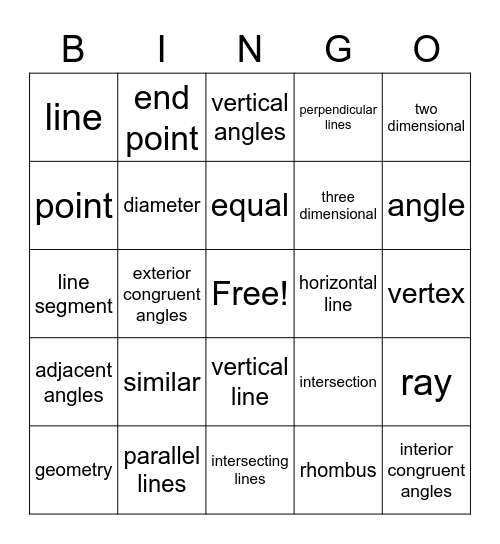# Geometry VocabularyThis bingo card has a free space and 24 words: similar, parallel lines, equal, geometry, two dimensional, line, point, end point, horizontal line, vertical line, three dimensional, line segment, intersection, ray, diameter, rhombus, vertex, vertical angles, angle, exterior congruent angles, interior congruent angles, adjacent angles, perpendicular lines and intersecting lines.

## Play Online